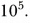# What is the ratio of SI units to CGS units of momentum? How do you measure the effect of an impulsive force on the body?

What is the ratio of SI units to CGS units of momentum? How do you measure the effect of an impulsive force on the body?

A ratio of SI units to CGS units of momentum is (kg m/s)/(g cm/s) i.e.,.